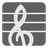The Greatest Common Divisor
Elementary+
DE English RU

"[The Euclidean algorithm] is the granddaddy of all algorithms, because it is the oldest nontrivial algorithm that has survived to the present day."
-- Donald Knuth, The Art of Computer Programming, Vol. 2: Seminumerical Algorithms, 2nd edition (1981).

The greatest common divisor (GCD), also known as the greatest common factor of two or more integers (at least one of which is not zero), is the largest positive integer that divides a number without a remainder. For example, the GCD of 8 and 12 is 4.

You are given an arbitrary number of positive integers. You should find the greatest common divisor for these numbers.

Input: An arbitrary number of positive integers.

Output: The greatest common divisor as an integer.

Example:

```greatestCommonDivisor(6, 4) == 2
greatestCommonDivisor(2, 4, 8) == 2
greatestCommonDivisor(2, 3, 5, 7, 11) == 1
greatestCommonDivisor(3, 9, 3, 9) == 3
```

Precondition:
1 < len(args) ≤ 10
all(0 < x ≤ 2 ** 32 for x in args)